Tutorials For Excel everyday with EVBA.info part 2[Free PDF ebook] - EBOOK VBA EXCELEVBA.info ( Ebook VBA Excel ) Welcome EVBA.info - Nothing Is Unable ... About Excel Tricks, Learning VBA Programming, Dedicated Software, Free Courses, Living Skills ...

## Thursday, January 16, 2020

Tutorials For Excel everyday with EVBA.info part 2[Free PDF ebook]

EVBA's Tutorials For Excel: Rounding Numbers By Fractions or Decimals
When rounding numbers to a particular decimal factor, you can express that rounding factor in your formula as either a fraction or as its decimal equivalent. In the pictures, the fraction one-eighth can be stated in a formula as 1/8 or by its decimal equivalent of .125.
Rounding up
Expressing a fraction, the formula in cell B4 copied to cell B8 is `=CEILING(A4,1/8)`
Expressing a decimal, the formula in cell D4 copied to cell D8 is `=CEILING(A4,0.125)`
Notice the results in range B4:B8 are the same as the results in range D4:D8.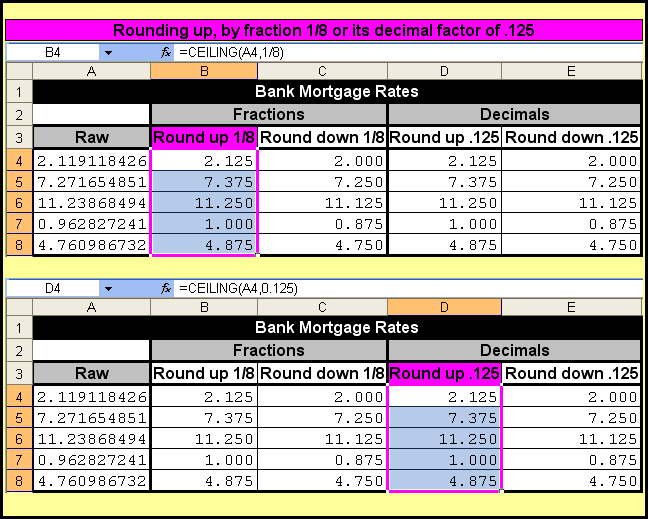Rounding down
Expressing a fraction, the formula in cell C4 copied to cell C8 is `=FLOOR(A4,1/8)`
Expressing a decimal, the formula in cell E4 copied to cell E8 is `=FLOOR(A4,0.125)`
Notice the results in range C4:C8 are the same as the results in range E4:E8.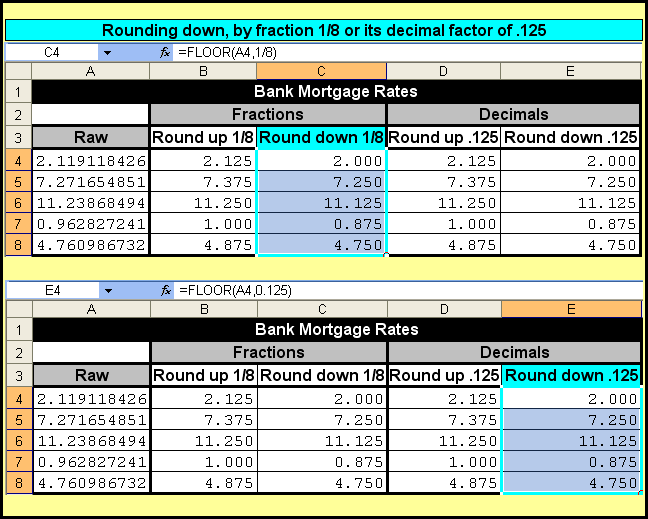Tom’s Tutorials For Excel: Evaluating Numbers as Being Whole or Decimal
There are times when you want to identify a number as being a whole number (such as 47) or a decimalized number (such as 23.5).
The picture shows three ways to apply this idea. You might want to identify a number as being whole or decimalized in a separate cell; or in the same cell, or disallow its attempt at entry.
In the TRUE or FALSE image, the formula in cell C3 is `=MOD(B3,1)=0` to identify decimal numbers.
In the Data Validation image, that same formula rule is used to disallow a decimal upon entry.
In the Conditional Formatting image, the formula to identify decimal numbers is `=MOD(B3,1)<>0`.
Tom’s Tutorials For Excel: Using VLOOKUP With MIN, MAX, and AVERAGE
You can nest a function as the `lookup_value` argument with `VLOOKUP`, to return an item relating to the `lookup_value` function. In the pictured example, `MIN``MAX`, and `AVERAGE` are nested to return the name of the salesperson associated with those functions.
The formula in cell D2 is `=VLOOKUP(MIN(A4:A22),A4:B22,2,0)`
The formula in cell E2 is `=VLOOKUP(MAX(A4:A22),A4:B22,2,0)`
The formula in cell F2 is `=VLOOKUP(AVERAGE(A4:A22),A4:B22,2,1)`
The `VLOOKUP` function’s fourth argument is `range_lookup`. For the `MIN` and `MAX` functions, the `range_lookup` is `0`, which could have also been written as `FALSE` or simply omitted altogether. This deals with your desire and expectation that an exact lookup value will be found. In this example, you know that a specific minimum and maximum number will be found in the list in column A.
However, you cannot be assured that the average of those numbers will specifically exist in the list. The average of the numbers in column A is 5218, not found in column A, so, the `1` (which could have been written as `TRUE`) was necessary in order for the formula to look up an approximate match.
Tom’s Tutorials For Excel: Doing Date Math on Text (non real) Dates
In yesterday’s tutorial, I showed how to place a date and time on separate lines in the same cell.
That example involved a formula with the `TEXT` function, meaning the date and time value in cell A3 was not a real date or real time, but a constant text value that was not unlike a text sentence such as you are reading now.
Common wisdom has it that you cannot perform mathematical functions on text dates. For example, if you try to add a 1 to the text value, you will return a `#VALUE!` error.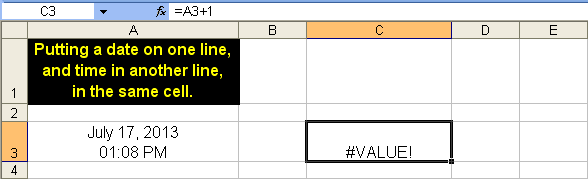However, you start to have some luck with a formula to return all to the left of `CHAR(10)`
`=(LEFT(A3,FIND(CHAR(10),A3)-1)+0)+1`
…which returns the serial number of (in this example) the date in cell C3 + 1, which is July 18, 2013.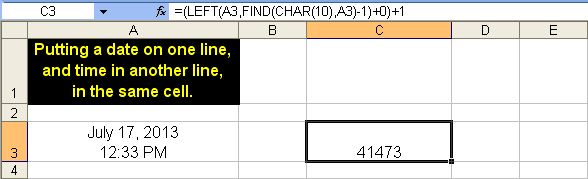In the Format Cells dialog box:
• Click onto the Number tab.
• In the Category list box, select Custom.
• In the Type field, enter your desired date format, such as `MMMM D, YYYY`
• Click the OK buttonTom’s Tutorials For Excel: Putting a Date on One Line and Time on Another Line in the Same Cell
Here’s a tip to place the date and time in the same cell, in separate lines.
The first step is to enter the formula
`=TEXT(NOW(),"MMMM D, YYYY")&CHAR(10)&TEXT(NOW(),"HH:MM AM/PM")`
The `CHAR(10)` notation refers to ascii character number 10, which is a carriage return character. In the cell, it looks like a small square, for example as pointed to by the red arrow.
Next,right-click the cell and select Format Cells from that pop-up menu.
In the Format Cells dialog box, click onto the Alignment tab. Click the dropdown arrow for the Horizontal field in the Text alignment section, and select Center.
Lastly, select the option for Wrap text, and click OK.
Tom’s Tutorials For Excel: Finding the Position of the First Integer in an Alphanumeric String
When you are faced with alphanumeric strings, such as those esoteric-looking serial numbers that represent a store’s stock items, here is how you can deal with parsing them based on the position of their first integer.
To find the position of the first integer, the formula in cell B2 and copied down to cell B13 is
`=MIN(FIND({0,1,2,3,4,5,6,7,8,9},A2&"0123456789"))`
Notice for example in cell B2, the formula returns 3. That is because cell A2 contains the alphanumeric string GS5453GDGD5. The first integer in that string is 5 and it is the third character (that is, in the third position) of that string.To extract everything to the left of the first-found integer, the formula in cell C2, copied to cell C13 is
`=LEFT(A2,MIN(FIND({0,1,2,3,4,5,6,7,8,9},A2&"0123456789"))-1)`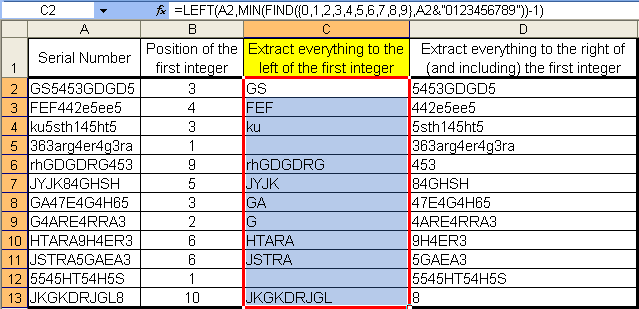A final example, to extract all characters to the right of — while including — the first found integer, the formula in cell D2 and copied down to cell D13 is
`=TRIM(REPLACE(A2,1,MIN(FIND({0,1,2,3,4,5,6,7,8,9},A2&"0123456789"))-1,""))`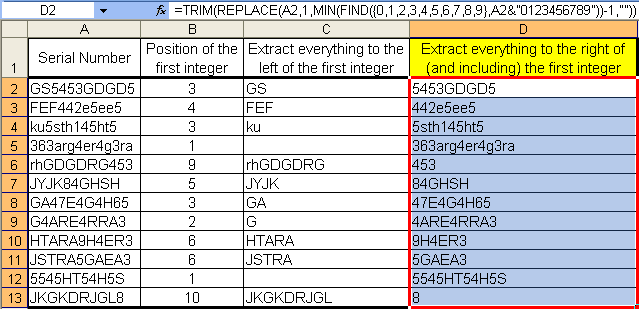Tom’s Tutorials For Excel: Finding the Number Closest to Zero
Here are two formulas, one to tell you the number closest to zero in a list, and the other to tell you the address of the cell holding that number. When you know a list does not contain a zero (if it did, you could simply do a `VLOOKUP` to find it), you can apply these array formulas as shown.
Recall, an array formula is applied to a cell by simultaneously pressing the `Ctrl+Shift+Enter` keys, not just `Enter`. The curly braces are not typed in by you; Excel places them automatically when the array formula is properly applied.
If you are unfamiliar with array formulas, see my video and explanation of arrays here.
Tom’s Tutorials For Excel: Returning a Value From Every Nth Cell
Here’s a formula to help you list (that is, return) the values from every (in this case) 6 cells. This is a useful method when your data is structured such that you know the incremental factor of rows that are in between cells that carry the data you want to separately list. It is especially handy when your source list is hundreds or thousand of rows deep.
In the pictured example, the formula in cell F2, which was copied down to cell F5 is
`=OFFSET(\$C\$2,ROW(C2)*6-6,0)`
Tom’s Tutorials For Excel: Adding and Subtracting Time in Hours Minutes and Seconds
Formula examples for hours, minutes, and seconds being added or subtracted from time.
Hours
Example to add 3 hours: `=\$B\$1+TIME(3,0,0)`
Example to subtract 3 hours: `=\$B\$1-TIME(3,0,0)`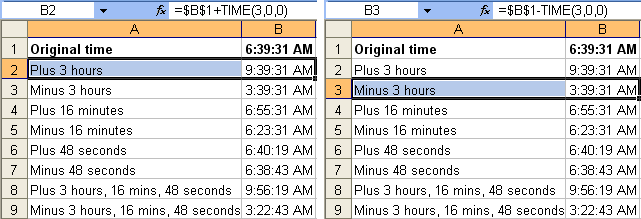Minutes
Example to add 16 minutes: `=\$B\$1+TIME(0,16,0)`
Example to subtract 16 minutes: `=\$B\$1-TIME(0,16,0)`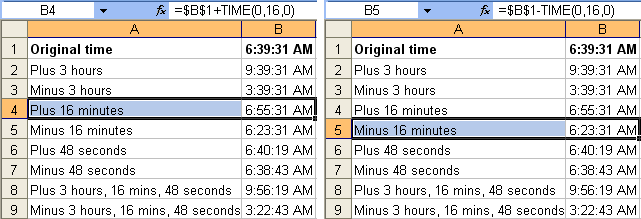Seconds
Example to add 48 seconds: `=\$B\$1+TIME(0,0,48)`
Example to subtract 48 seconds: `=\$B\$1-TIME(0,0,48)`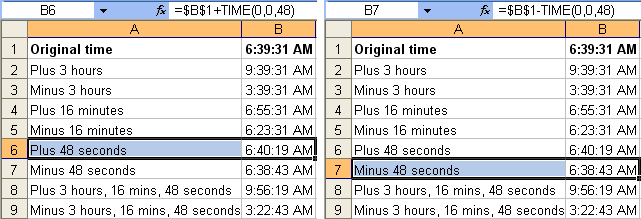Combination of Hours, Minutes, and Seconds
Example to add 3 hours, 16 minutes, and 48 seconds: `=\$B\$1+TIME(3,16,48)`
Example to subtract 3 hours, 16 minutes, and 48 seconds: `=\$B\$1-TIME(3,16,48)`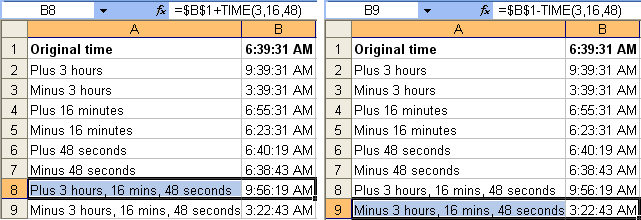Tom’s Tutorials For Excel: Validating an Entry as a Real Date
One way to verify that a bona fide date is being entered into a cell is to use Data Validation.
In the pictured example, dates are being entered into a list in column E. The attempted entry in cell E6 is being rejected because it is not a date.
Step 1 of 4: Before you enter dates, set up your worksheet:
• Start by selecting the range where dates will be entered.
• Then, from your keyboard press `Alt+D+L` to show the Data Validation dialog box.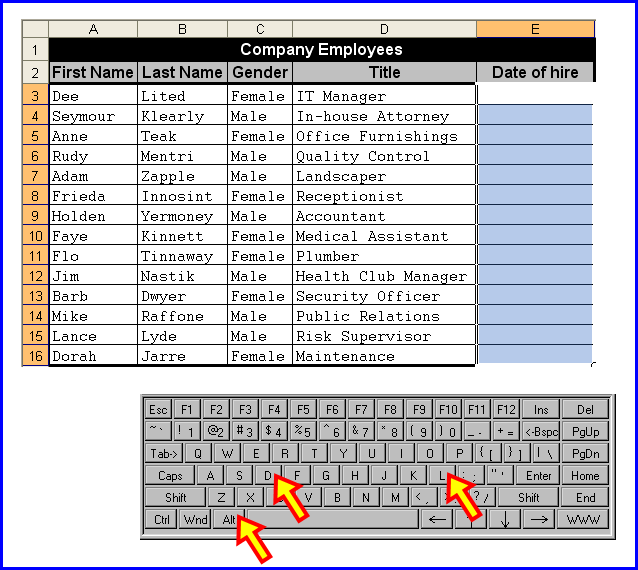Step 2 of 4: In the Data Validation dialog box:
• Go to the Settings tab.
• Click the drop-down arrow for the Allow field.
• In the list of allowable settings, click to select Custom.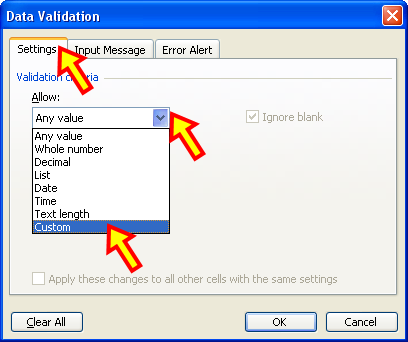Step 3 of 4: Still in the Data Validation dialog box:
In the Formula field, enter your formula rule.
Notice the range being Data Validated (the selected range in Step 1) is E3:E16.
The Data Validation formula being used, with cell E3 as the active cell in that selection, is
`=AND(ISNUMBER(E3),LEFT(CELL("format",E3),1)="D")`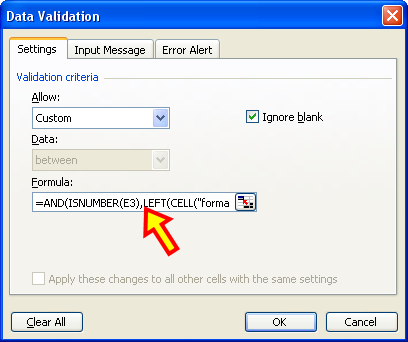Step 4 of 4: Still in the Data Validation dialog box:
• Click onto the Error Alert tab.
• Click to select the option for (that is, click to put a check in the box next to) “Show error alert after invalid data is entered”.
• For the Style field, click the drop-down arrow and select “Stop”.
• In the Title field, enter a short headline such as you see here, and as you see in practice in the picture at the top of this tutorial.
• In the Error message field, enter an informative explanation as to why the attempted entry is being rejected, and what the user should do to correct that action.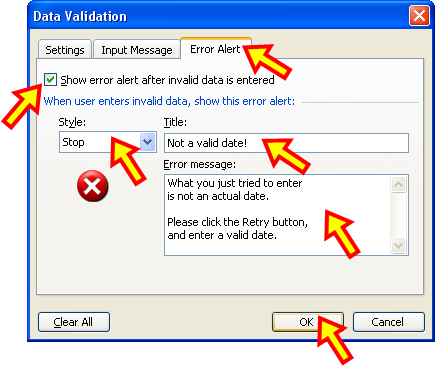📤You download App EVBA.info installed directly on the latest phone here : https://www.evba.info/p/app-evbainfo-setting-for-your-phone.html?m=1